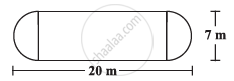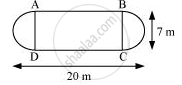Share

# The Shape of a Garden is Rectangular in the Middle and Semi Circular at the Ends as Shown in the Diagram. Find the Area and the Perimeter of the Garden [Length of Rectangle is 20 − (3.5 + 3.5) Metres] - CBSE Class 8 - Mathematics

ConceptIntroduction of Mensuration

#### Question

The shape of a garden is rectangular in the middle and semi circular at the ends as shown in the diagram. Find the area and the perimeter of the garden [Length of rectangle is 20 − (3.5 + 3.5) metres]#### Solution

Length of the rectangle = [20 − (3.5 + 3.5)] metres = 13 m

Circumference of 1 semi-circular part = π=(22/7 xx 3.5)m = 11 m

Circumference of both semi-circular parts = (2 × 11) m = 22 mPerimeter of the garden = AB + Length of both semi-circular regions BC and

DA + CD

= 13 m + 22 m + 13 m = 48 m­

Area of the garden = Area of rectangle + 2 × Area of two semi-circular regions

[(13xx7)+2xx1/2xx22/7xx(3.5)^2]m^2

= (91+38.5)m^2

= 129.5 m^2

Is there an error in this question or solution?

#### APPEARS IN

NCERT Solution for Mathematics Textbook for Class 8 (2018 to Current)
Chapter 11: Mensuration
Ex. 11.10 | Q: 3 | Page no. 171
Solution The Shape of a Garden is Rectangular in the Middle and Semi Circular at the Ends as Shown in the Diagram. Find the Area and the Perimeter of the Garden [Length of Rectangle is 20 − (3.5 + 3.5) Metres] Concept: Introduction of Mensuration.
S